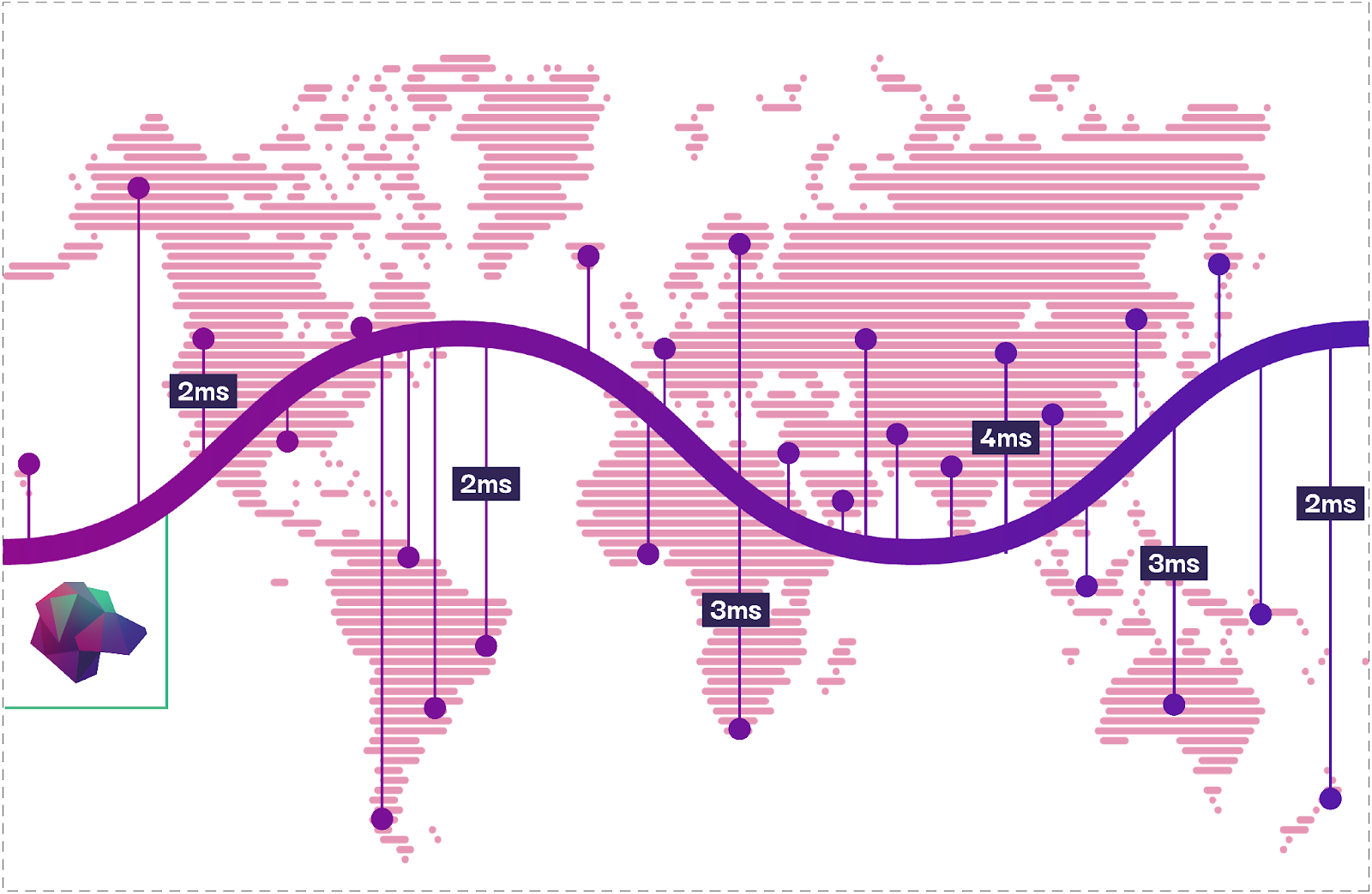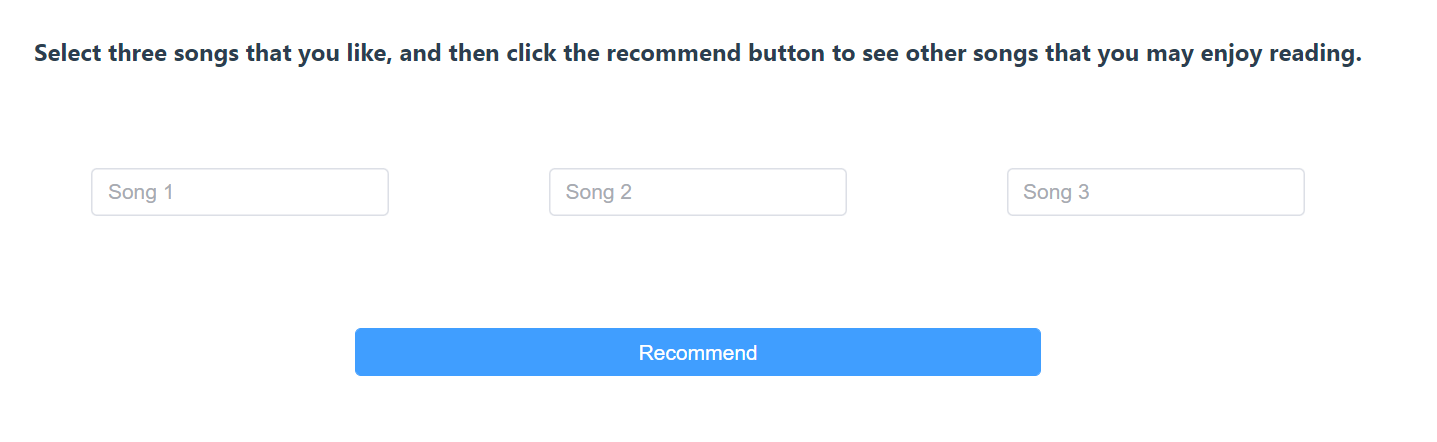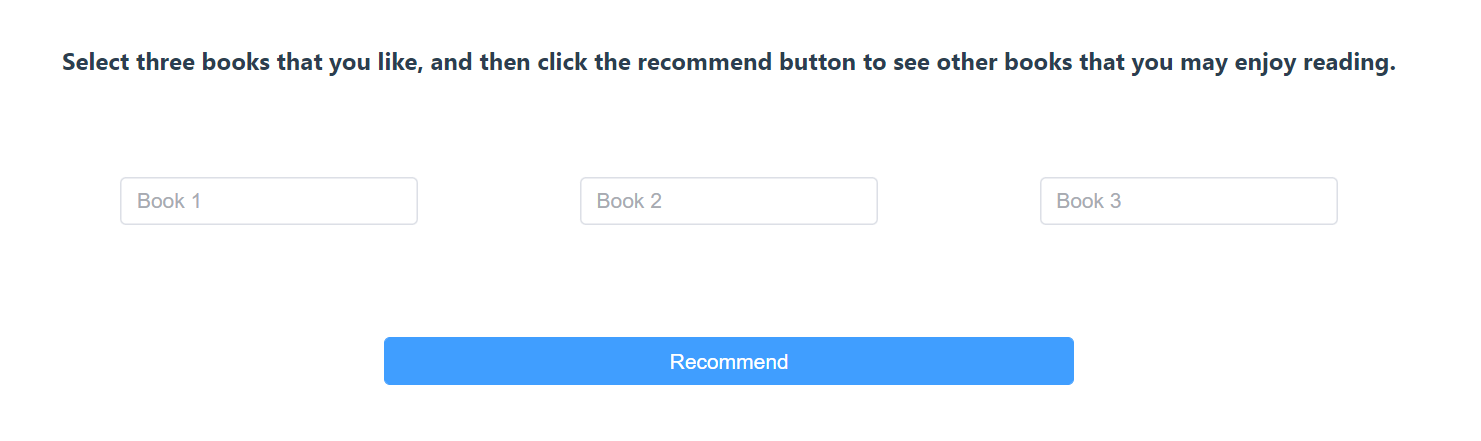# How to Build a Machine Learning Recommendation Engine w/ TensorFlow & HarperDB

This article explains how to create a recommendation system using HarperDB and Custom Functions.

With HarperDB we are able to deploy a machine learning model to servers located on the edge of the Internet to provide users with content recommendations. We have two examples to demonstrate how this can be done: a book recommender and a song recommender.## An Intro to Recommendation Systems

Recommendation engines are the most universally used type of machine learning. We interact with them everywhere, and it has become the driving force for how we explore new content. And while some are less than perfect, even the least of them are miles ahead of the previous methods we had available to find new things.Take shows and movies for example. Do you remember the preview channel… staring at it for hours, trying to guess which titles would be worth further exploration? The same is true for audio content, where we were limited to radio stations to find new music. And books were even more difficult. I’d walk along the bookstore aisles picking random books to read their summary before finally finding one to take a chance on.

All of these methods did work, but they limited us. We could only truly explore the content which was made available by the system at hand – such as the preview channel, radio station, and bookstore shelves. But there is so much more content out there, especially today. So instead of having to choose from the limited options presented by the Best Sellers section at the bookstore, how does one find new content that’s based on their individual interests?

Recommendation Systems!## What is a Recommendation System? How do they work?

Recommendation systems are exactly what they sound to be; a system that provides recommendations. You give it an idea about what you like, and it points you in the direction of other things you might like.

There are many ways to set up such a system. For example, if we’re talking about products, a system could be built that finds all of the users who bought the same product as you, and then displays the most common products among that group. This could be expanded by adding more items to the input. Such as moving from a single product to three products that you bought, grouping together all of the users who also bought those three items, and then displaying the most common other products among that group.

What if we’re including ratings with the items of interest? For example, if many people are watching one particular movie, does that automatically mean it’s a good movie to recommend? And if not, then how would we prevent it from appearing in the recommendations? When including ratings and reviews, we could set up a filter to remove any content with a bad review to ensure the group of items we’re aggregating always contains positive content. This is a great starting point. It works for many cases where you have a dataset of items and user interactions with those items.

The challenging part arises from the evolving nature of content. How would a song, book, or product that’s new get into the output of the recommendation system?## Using Machine Learning

While we could keep adding manual filters to the content by sorting the ratings and weighting interactions with new items, there would be areas where we fall short. Especially when creating binary gates such as a positive/negative rating threshold to determine which items are included in the output.

This is where machine learning takes over. Using libraries such as TensorFlow Recommenders with Keras models, it’s easy to shape the data in ways that will allow the items and users to be viewed and compared in a multidimensional perspective. Qualitative features such as item categories and user profile attributes can be mapped into mathematical concepts that can be quantitatively compared with one another, ultimately providing new insights and better recommendations.

Using a machine learning pipeline like this also allows the models to be continuously trained by taking the results of the previous model, the successfulness of those recommendations to drive user interactions, and using that data to create better models. I recently wrote an article about using TensorFlowJS and HarperDB for Machine Learning if you’d like to learn more.

## HarperDB Recommenders

One of the awesome features of living on the edge is the ability to connect users to new insights with low latency and minimal traffic. With HarperDB Custom Functions, we can deploy a model to multiple nodes in a cluster, allowing the closest and most available to return the result to the user.

Let’s look at two example projects to demonstrate how this would look when providing recommendations to a user based on provided content to create a song recommender and a book recommender.

To ensure the examples are reusable and straightforward, these projects are the frontside of the process, where we’re serving an already trained model. In production we would connect a second piece to this puzzle where we track user interactions in a central location, train new models, and deploy them back out to the edge with HaperDB.

## HarperDB Song RecommenderGithub repo: HarperDB/song-recommender

In our Song Recommender example, a user can find three of their favorite songs in the UI, and then the recommendation system returns other songs they’re likely to enjoy.

There’s a dataset called The Million Song Dataset that contains very detailed information on over one million songs, ranging from audio analysis to the location of the artist.

There’s a nice subset of that data called The Echo Nest Taste Profile Subset which is a list of users, songs, and play counts. Each row in the data contains a userid, songid, and playcount. This is what we used to build the model for this project.### Training the Model

We created a Two-Tower model, which is a design where two latent vectors are created, compared during training to shape the embedding layers, and compared during inference to find the best match.

Tower One is the Query Tower. This is essentially the input to the equation, which in this case is three songs that the user selects.

Tower Two is the Candidate Tower. In this case it’s the users in the dataset.

We find the user in the dataset most similar to the user using the UI, and then provide their most played songs as recommendations.

To look at the training specifics, here’s the training notebook.

The basic steps that are taken are as follows:

1. Find the most played songs for each user
2. Create a query/candidate pair of three of those songs and the user ([songs], user)
3. Build two models and setup TFRS metrics for training
4. Feed the query/candidate pair into the model
5. When training is complete, create a new model that wraps the above two
6. Apply the BruteForce method to extract the best candidate match provided an input

Generating the Data

```# create a dictionary of inputs and outputs
dataset = {'songs': [], 'user': []}
for user, songs in users_songs.items():
for _ in range(len(songs) * 5):
# randomly select n_songsns_in from the user's isbns
selected_songs = np.random.choice(songs, n_songs_in)
# add them to the inputs
dataset['songs'].append(selected_songs)
# add the user to the output
dataset['user'].append(user)
```

Building the Models

```# create the query and candidate models
n_embedding_dimensions = 24

## QUERY
songs_in_in = tf.keras.Input(shape=(n_songs_in))
songs_in_emb = tf.keras.layers.Embedding(n_songs+1, n_embedding_dimensions)(songs_in_in)
songs_in_emb_avg = tf.keras.layers.AveragePooling1D(pool_size=3)(songs_in_emb)
query = tf.keras.layers.Flatten()(songs_in_emb_avg)
query_model = tf.keras.Model(inputs=songs_in_in, outputs=query)

## CANDIDATE
user_in = tf.keras.Input(shape=(1))
user_emb = tf.keras.layers.Embedding(n_users+1, n_embedding_dimensions)(user_in)
candidate = tf.keras.layers.Flatten()(user_emb)
candidate_model = tf.keras.Model(inputs=user_in, outputs=candidate)
```

```# TFRS TASK SETUP
candidates = dataset.batch(128).map(lambda x: candidate_model(x['user']))
metrics = tfrs.metrics.FactorizedTopK(candidates=candidates)

## TFRS MODEL CLASS
class Model(tfrs.Model):
def __init__(self, query_model, candidate_model):
super().__init__()
self._query_model = query_model
self._candidate_model = candidate_model

def compute_loss(self, features, training=False):
query_embedding = self._query_model(features['songs'])
candidate_embedding = self._candidate_model(features['user'])

## COMPILE AND TRAIN MODEL
model = Model(query_model, candidate_model)
# load model weights - this is to resume training

model.fit(dataset.repeat().shuffle(300_000).batch(4096), steps_per_epoch=50, epochs=30, verbose=1)
```

### Deploying the Model

To deploy this model, the final step in the above notebook is converting it to TensorFlowJS.

From there, we add it to the Custom Function’s directory along with the logic in recommend.js which converts the user’s three favorite songs into tensors which are provided to the model.

The output of the model is a reference to the most similar user to the one using the UI.

The top songs for that user are then returned and displayed in the UI as our recommendations.

Creating an input tensor and getting results

``` if (!this.model) {
const modelPath = path.join(__dirname, '../', 'tfjs-model', 'model.json');
}

const inputTensor = tf.tensor([songIdxs], [1, 3], 'int32')
const results = this.model.execute(inputTensor)
const r0 = await results.data()
```

## HarperDB Book RecommenderGithub repo: HarperDB/book-recommender

In our Book Recommender example, a user can find three of their favorite books in the UI, and then the recommendation system returns other books they’re likely to enjoy.

There’s a dataset on Kaggle that includes a list of users, books, and ratings for about 250,000 different titles.### Training the Model

We again created a Two-Tower model, which is a design where two latent vectors are created, compared during training to shape the embedding layers, and compared during inference to find the best match.

Tower One is the Query Tower. This is essentially the input to the equation, which in this case is three books that the user rated highly (a 5 and above out of 10).

Tower Two is the Candidate Tower. In this case it’s the users in the dataset.

We find the user in the dataset most similar to the user using the UI, and then provide their highest rated books as recommendations.

To look at the training specifics, here’s the training notebook.

The basic steps that are taken are as follows:

1. Find the top rated books for each user
2. Create a query/candidate pair of three of those books and the user ([books], user)
3. Build two models and setup TFRS metrics for training
4. Feed the query/candidate pair into the model
5. When training is complete, create a new model that wraps the above two
6. Apply the BruteForce method to extract the best candidate match provided an input

Generating the Data

```# create a dictionary of inputs and outputs
dataset = {'isbns': [], 'user': []}
for user_id, isbns in user_isbns.items():
# use 5x the number of isbns gathered for the user
# this ensures a larger amount of training data
for _ in range(len(isbns) * 5):
# randomly select n_isbns_in from the user's isbns
selected_isbns = np.random.choice(isbns, n_isbns_in)
# add them to the inputs
dataset['isbns'].append(selected_isbns)
# add the user to the output
dataset['user'].append(user_idxs[user_id])
```

Building the Models

```# create the query and candidate models
n_embedding_dimensions = 24

## QUERY
isbns_in_in = tf.keras.Input(shape=(n_isbns_in))
isbns_in_emb = tf.keras.layers.Embedding(n_isbns+1, n_embedding_dimensions)(isbns_in_in)
isbns_in_emb_avg = tf.keras.layers.AveragePooling1D(pool_size=3)(isbns_in_emb)
query = tf.keras.layers.Flatten()(isbns_in_emb_avg)
query_model = tf.keras.Model(inputs=isbns_in_in, outputs=query)

## CANDIDATE
isbns_out_in = tf.keras.Input(shape=(1))
isbns_out_emb = tf.keras.layers.Embedding(n_users+1, n_embedding_dimensions)(isbns_out_in)
candidate = tf.keras.layers.Flatten()(isbns_out_emb)
candidate_model = tf.keras.Model(inputs=isbns_out_in, outputs=candidate)
```

```# TFRS TASK SETUP
candidates = dataset.batch(128).map(lambda x: candidate_model(x['user']))
metrics = tfrs.metrics.FactorizedTopK(candidates=candidates)

## TFRS MODEL CLASS
class Model(tfrs.Model):
def __init__(self, query_model, candidate_model):
super().__init__()
self._query_model = query_model
self._candidate_model = candidate_model

def compute_loss(self, features, training=False):
query_embedding = self._query_model(features['isbns'])
candidate_embedding = self._candidate_model(features['user'])

## COMPILE AND TRAIN MODEL
model = Model(query_model, candidate_model)
# load model weights - this is to resume training

model.fit(dataset.repeat().shuffle(300_000).batch(4096), steps_per_epoch=50, epochs=30, verbose=1)
```

### Create the BruteForce Comparison

```# create the index model to lookup the best candidate match for a query
index = tfrs.layers.factorized_top_k.BruteForce(model._query_model)
index.index_from_dataset(
tf.data.Dataset.zip((
dataset.map(lambda x: x['user']).batch(100),
dataset.batch(100).map(lambda x: model._candidate_model(x['user']))
))
)
for features in dataset.shuffle(2000).batch(1).take(1):
print('isbns', features['isbns'])
scores, users = index(features['isbns'])
print('recommended users', users)
```

### Deploying the Model

To deploy this model, the final step in the above notebook is converting it to TensorFlowJS.

From there, we add it to the Custom Function’s directory along with the logic in recommend.js which converts the user’s three favorite books into tensors which are provided to the model.

The output of the model is a reference to the most similar user to the one using the UI.

The top rated books for that user are then returned and displayed in the UI as our recommendations.

## Recap

And there you have it, that’s how you can create a recommendation system with HarperDB Custom Functions.

These machine learning models were pre-trained for these examples, as they do take 12+ hours to reach their most accurate state. In a production environment, there would most likely be a single instance responsible for continuously training the model and distributing it out to the other nodes on the edge.

Go ahead and launch a HarperDB instance with Custom Functions where you can pull in one of these repos and get recommendations for new songs and books to check out. If you get an interesting result that you enjoy, please let us know!